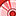# QlikView App Dev

Discussion Board for collaboration related to QlikView App Development.

Announcements
Join this live chat April 6, 10AM EST - QlikView to Qlik Sense REGISTER
cancel
Showing results for
Search instead for
Did you mean:Contributor III

## Percent of Total Dimension

Hello,

I have a pivot table (the first one) with the following dimensions: Location, Dimension and Line and three expressions:

1. The Total Amount by dimensions

2. %: the percent between each line and the R line.

3. AVG %: the percent between each line and the R line but without location selected.

I made the first and the second expressions.

One problem is that the second expression isn't calculated at the Total, and the biggest problem is that I can't make the third expression to ignore the location. The AVG % should be exactly like the AVG % from the second table, no matter what location is in the first table.

I attached my qvw file.

Can you help me?

Thank you!

Labels (2)

• ### percent

1 Solution

Accepted SolutionsMVP

Hi, for 2nd expession you can use:

=sum(Amount)
/sum(TOTAL <Dimension,Location> {<Line={"R1","R2"}>} Amount)

And for the 3rd:

=sum(TOTAL <Dimension, Line> Amount)
/sum(TOTAL <Dimension> {<Line={"R1","R2"}>} Amount)

2 RepliesMVP

Hi, for 2nd expession you can use:

=sum(Amount)
/sum(TOTAL <Dimension,Location> {<Line={"R1","R2"}>} Amount)

And for the 3rd:

=sum(TOTAL <Dimension, Line> Amount)
/sum(TOTAL <Dimension> {<Line={"R1","R2"}>} Amount)Contributor III
Author

It's working. Thank you!